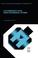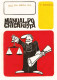### Computational Electromagnetism

#### Course Description

Problems with complex geometry and material properties often arise in development and design of electromagnetic devices. Application of analytical procedures introduced in the course "Electromagnetic Fields" often results with unsatisfactory accuracy of results, and application of numerical method is necessary. Main topics are: Maxwell s equations, electromagnetic potentials, wave equations, integrals of potentials and fields. Finite element method, computation of electric fields and capacitances. Nonlinear problems, calculation of magnetic fields and inductances. Quasistatic problems, calculation of skin effect and eddy currents. Application in electromagnetic compatibility, modeling and analysis of electric devices. Method of moments, calculation of low-frequency electric and magnetic field, application in analysis of human exposure to electromagnetic fields.

#### General Competencies

Understanding of theoretical foundations of electromagnetism, and of numerical approach to solution of practical problems.

#### Learning Outcomes

1. explain electromagnetic field theory
2. apply of potentials in solution of electromagnetic field problems
3. explain fundamental properties of finite element method
4. explain fundamental properties of method of moments
5. develop models for application of numerical methods
6. apply numerical methods to solution of practical problems
7. identify aplicability of results of numerical calculations
8. use commercial application software with insight into fundamental properties and limitations

#### Forms of Teaching

Lectures

Exams

Laboratory Work

Experimental Exercises

Consultations

Seminars

Continuous Assessment Exam
Laboratory Exercises 0 % 8 % 0 % 8 %
Homeworks 0 % 8 % 0 % 8 %
Quizzes 0 % 4 % 0 % 4 %
Seminar/Project 0 % 30 % 0 % 30 %
Mid Term Exam: Written 0 % 20 % 0 %
Final Exam: Oral 30 %
Exam: Written 0 % 20 %
Exam: Oral 30 %

#### Week by Week Schedule

1. Maxwell's equations in time and frequency domain.
2. Electromagnetic potentials, wave equations.
3. Integrals of potentials and fields.
4. Finite element method, functional.
5. Weighted residual method. Poisson's equation.
6. Approximation of domain geometry, approximation of potential function.
7. Assembling and solution of equation system, postprocessing.
8. Mid-term exam
9. Lab: solving of static electric fields in electrical devices (calculation of capacitances, shielding).
10. Lab: non-linear problems, calculation of magnetic field (calculation of inductances, shielding).
11. Lab: quasistatic problems (eddy currents, skin effect, shielding).
12. Electromagnetic compatibility, numerical models.
13. Method of moments. Integral formulation of electric and magnetic field problems.
14. Lab: calculation of low-frequency electric and magnetic field.
15. Analysis of human exposure to electromagnetic fields, examples.

#### Study Programmes

Control Engineering and Automation (profile)
Recommended elective courses (3. semester)
Electronic and Computer Engineering (profile)
Recommended elective courses (3. semester)
Electronics (profile)
Recommended elective courses (3. semester)
Wireless Technologies (profile)
Recommended elective courses (3. semester)

#### Literature

Z. Haznadar, Ž. Štih (1997.), Elektromagnetizam 1 i 2, Školska knjigaZ. Haznadar, Ž. Štih (2000.), Electromagnetic Fields, Waves and Numerical Methods, IOS PressG. Dhatt, G. Thouzot (1985.), The Finite Element Method Displayed;, John Wiley & Sons

#### General

ID 34494
Winter semester
4 ECTS
L3 English Level
L1 e-Learning
30 Lectures
0 Exercises
0 Laboratory exercises
0 Project laboratory